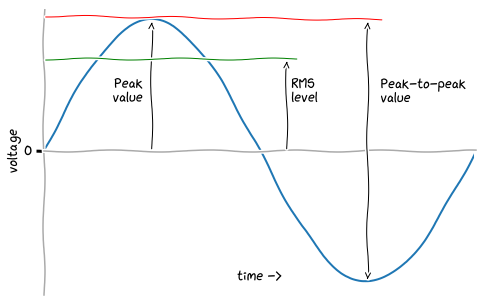# Mains voltage• UK mains voltage is 230V RMS value
• RMS means root mean square
• the RMS value is calculated from a peak value
• the peak value is the same as the amplitude of the wave
• the peak value isn’t the same as the peak-to-peak value
• the RMS value depends on the shape of the wave
• for a sinusoid, the RMS value is about `0.707` of its peak value
• the coefficient comes from `1/√2 ≈ 0.707`

Thus:

• taking the RMS value of `230V`
• `230V / 0.707 ≈ 325V`; that’s the peak value (or the amplitude)
• the peak-to-peak value is twice that, at `650V`